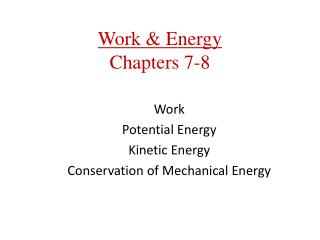DownloadDownload PresentationWork & Energy Chapters 7-8

# Work & Energy Chapters 7-8

Télécharger la présentation## Work & Energy Chapters 7-8

- - - - - - - - - - - - - - - - - - - - - - - - - - - E N D - - - - - - - - - - - - - - - - - - - - - - - - - - -
##### Presentation Transcript

1. Work & EnergyChapters 7-8 Work Potential Energy Kinetic Energy Conservation of Mechanical Energy

2. 7-1 Work Done by a Constant Force The definition of work, when the force is parallel to the displacement: (7-1) SI unit: newton-meter (N·m) = joule, J

3. 7-1 Work Done by a Constant Force If the force is at an angle to the displacement: (7-3)

4. 7-1 Work Done by a Constant Force If there is more than one force acting on an object, we can find the work done by each force, and also the work done by the net force: (7-5)

5. 7-3 Work Done by a Variable Force If the force is constant, we can interpret the work done graphically:

6. 7-3 Work Done by a Variable Force If the force takes on several successive constant values:

7. 7-3 Work Done by a Variable Force The force needed to stretch a spring an amount x is F = kx. Therefore, the work done in stretching the spring is (7-8)

8. 7-2 Kinetic Energy and the Work-Energy Theorem After algebraic manipulations of the equations of motion, we find: Therefore, we define the kinetic energy: (7-6)

9. 7-2 Kinetic Energy and the Work-Energy Theorem Work-Energy Theorem: The total work done on an object is equal to its change in kinetic energy. (7-7)

10. 7-4 Power Power is a measure of the rate at which work is done: (7-10) SI unit: J/s = watt, W 1 horsepower = 1 hp = 746 W

11. 8-1 Conservative and Nonconservative Forces Work done by gravity on a closed path is zero:

12. 8-1 Conservative and Nonconservative Forces Work done by friction on a closed path is not zero:

13. 8-2 The Work Done by Conservative Forces Gravitational potential energy:

14. 8-2 The Work Done by Conservative Forces If we pick up a ball and put it on the shelf, we have done work on the ball. We can get that energy back if the ball falls back off the shelf; in the meantime, we say the energy is stored as potential energy. (8-1)

15. 8-2 The Work Done by Conservative Forces (8-4) Springs:

16. 8-3 Conservation of Mechanical Energy Definition of mechanical energy: (8-6) Using this definition and considering only conservative forces, we find: Or equivalently: